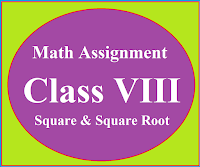## Posts

Showing posts from October, 2020

### Math Assignment Class VIII | Square & Square RootMath Assignment  Class VIII | Square & Square Root Download or Print free  assignment with answer key  for   Class  8 Squares and  Square Roots.   Important and extra questions that cover all topics of square and square root and is useful and helpful for the students. Math Assignment  Class VIII | Square & Square Root LEVEL -1

### Math Assignment | Class XII | Ch-10 | Vector AlgebraMaths Assignment Class 12 Vector Algebra Chapter 10 Extra questions important for the examination. Maths assignment  on vector algebra for complete knowledge of the concept. Important steps that students need to do First of all students should learn and  revise all basic concepts and formulas of vector algebra. Revise Chapter 10 vector algebra  NCERT book of Mathematics. Revise all examples of chapter 10 of  NCERT book. Now start the Assignment. Assignment on Vector Algebra   Question 1  :$Show\;that\; the \; points\; with\; P. V.\; \vec{a}-2\vec{b}+3\vec{c},\; \;-2\vec{a}+3\vec{b}+2\vec{c}\; and$$-8\vec{a}+13\vec{b}\; are\; collinear\; vectors.$Hint: Let given vectors are the position vectors of point A, B and C. Find vector AB and AC. If one vector is the scalar multiple of the other then they are called parallel vectors. Also these are co-initial vectors so these vector are called collinear vectors.   Question 2 :  If A is a point (1, -2) a